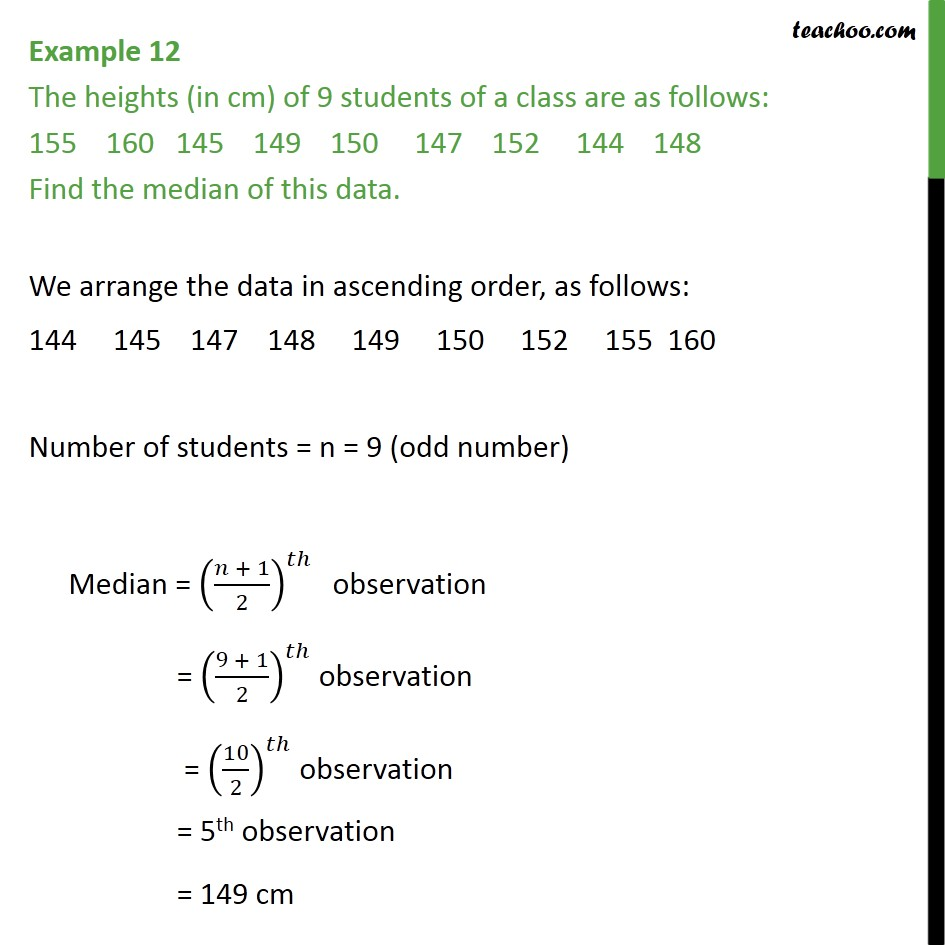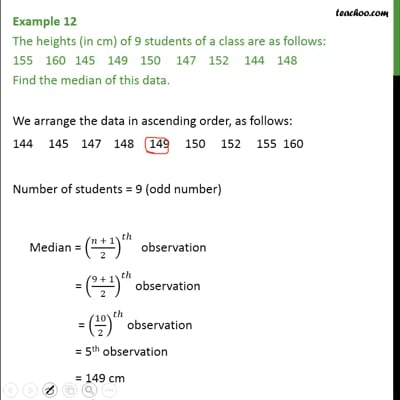Median

Chapter 14 Class 9 Statistics
Concept wiseThis video is only available for Teachoo black users

Introducing your new favourite teacher - Teachoo Black, at only ₹83 per month

### Transcript

Example 12 The heights (in cm) of 9 students of a class are as follows: 155 160 145 149 150 147 152 144 148 Find the median of this data. We arrange the data in ascending order, as follows: 144 145 147 148 149 150 152 155 160 Number of students = n = 9 (odd number) Median = ((𝑛 + 1)/2)^𝑡ℎ " obs"ervation = ((9 + 1)/2)^𝑡ℎ observation = (10/2)^𝑡ℎ observation = 5th observation = 149 cm